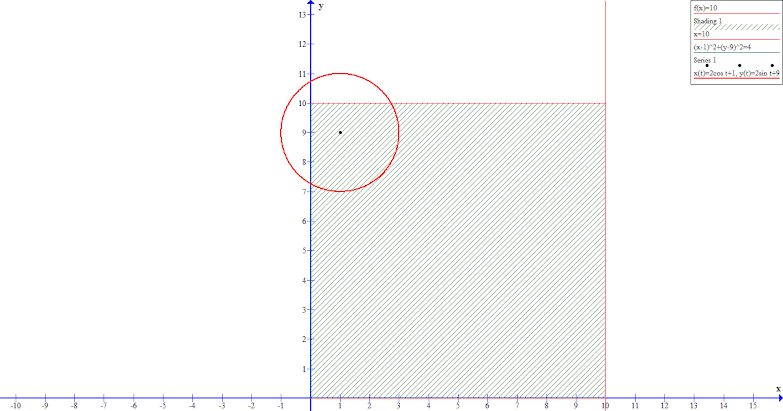# Calculate area of a specific part of a circle?

I am trying to calculate the area of a specific region inside a circle.

The region, in this case, is the green area inside the circle.

The given material is the equation, radius and the center of the circle and the equation of the lines forming the region in which the required area of circle has to be calculated.• Find out the intersections of the circle and rectangle borders, then join each of the two intersections to the centre of circle. Now you have to find the area of a sector and two triangles. – peterwhy Jan 5 '14 at 12:58
• @peterwhy And how, in that case, do I calculate the angle formed by the sector on the center of the circle? – Ashish Pahwa Jan 5 '14 at 13:04
• By trigonometry, the easiest way to explain is by cosine rule. But by observation the angle is $30^\circ+90^\circ+30^\circ$... – peterwhy Jan 5 '14 at 13:09

I'm assuming that you have to solve this problem many times and want a foolproof formula avoiding cases.

I'm referring to the following figure, where it is tacitly assumed that $u^2+v^2<r^2$.One has $$A=\bigl(u,-\sqrt{r^2-u^2}\bigr), \qquad B=\bigl(\sqrt{r^2-v^2},v\bigr)$$ and $$\phi_A:={\rm Arg}(A)=-{\pi\over2}+\arcsin{u\over r},\qquad \phi_B:={\rm Arg}(B)=\arcsin{v\over r}\ .$$ Now according to Green's area formula one has \eqalign{{\rm area}(\Omega)&={1\over2}\int\nolimits_{\partial\Omega}(x\ dy-y\ dx)\cr &={v\over2}\bigl(\sqrt{r^2-v^2}-u\bigr)-{u\over2}\bigl(v+\sqrt{r^2-u^2}\bigr)+{r^2\over2}(\phi_B-\phi_A)\ .\cr} The last term was obtained using the parametrization $$\phi\mapsto(r\cos\phi,r\sin\phi)\quad(\phi_A\leq\phi\leq\phi_B)\ .$$

Decompose your area into polygons and circular segments, then sum their areas.

(Because of my laziness,) Move the origin to the centre of circle. The circle intersects the horizontal edge at \begin{align*} x^2 + 1^2 =& 2^2\\ x =& \sqrt3 \end{align*} and $y=1$. Similarly the circle also intersects the rectangle at $(-1, -\sqrt3)$.

The first intersection makes an angle with positive $x$-axis $\arctan\frac1{\sqrt3} = 30^\circ$, and the second intersection makes an angle with positive $x$-axis $\arctan\sqrt3-180^\circ = -120^\circ$. So the angle of sector is $150^\circ$.

So area of sector is $$\pi\times2^2\times\frac{150}{360} = \frac{5\pi}3$$